# 2023 Fantasy Football Player Rankings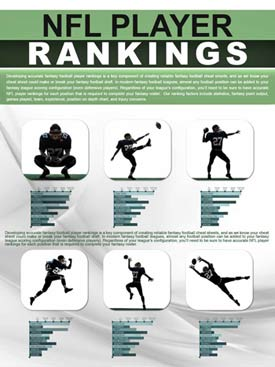Developing accurate fantasy football player rankings is a key component of creating reliable fantasy football cheat sheets, and as we know your cheat sheet could make or break your fantasy football draft. In modern fantasy football leagues, almost any football position can be added to your fantasy league scoring configuration (even defensive players).

Regardless of your league’s configuration, you’ll need to be sure to have accurate NFL player rankings for each position that is required to complete your fantasy roster. Otherwise, how would you know how to make picks for your fantasy football draft boards as the season approachs? Here are some factors we consider when developing our fantasy football player rankings:

• Statistics- Obviously the stats from the previous season are most critical but as the season progresses current statistics become more relevant.
• Fantasy Point Output- Understand how each player’s statistics translate to point output based on your fantasy league configuration. Just as statistic/point-values vary from league to league, a player's ranking will likewise rise or fall based on league settings.
• Games Played- The number of games a player actually played in during the previous season is an oft-forgotten statistic. Would you rather draft a player that put up 80 fantasy points in 16 games, or a player who put up 60 fantasy points in 8 games? This is why FPPG output is a key statistic to follow (and also why it is integrated into all of our sheets by default).
• Team- The team a player plays for should definitely factor into their ranking, especially if the player has changed teams. Those teams with high-powered offenses will have players who put up more fantasy points, so remember to factor in the team when you configure your rankings.
• Experience- The factor that experience plays in your NFL player rankings is heavily dependent on the position being analyzed; a productive kicker will have a much longer career than a productive running back. Compare each player’s stats to their experience to ensure they are not on the downside of their career.
• Position on Depth Chart- As training camp battles progress, a clearer picture of each team’s depth chart should arise. Know which players have cemented a starting job and which players are still battling to move up the depth chart.
• Injury Concerns– Football players are constantly battling injuries, but some injuries are worse than others. Those players with injury concerns should slide down your player rankings; how far they slide will depend on the severity (and sometimes the frequency) of their injury.

There are many different factors to consider when configuring your 2023 NFL player rankings. The key to success is examining the relevant factors and properly applying them to the position and player being analyzed. We hope that the rankings provided will help you create reliable and accurate fantasy football cheat sheets in preparation for your 2023 fantasy football draft. Below are our top 5 players at each position named in the standard fantasy football scoring system. To view all rankings for a particular position please click the links above.

## Top 10 Quarterbacks

(view all)
1Patrick Mahomes #13
 KC Chiefs, 5 years exp
GAM=17, PAYD=5250, PATD=41, RUCA=61, RUYD=358, RUTD=4, INT=12, FUM=0, FPPG=28.9, TFP=492
2Josh Allen #13
 BUF Bills, 5 years exp
GAM=16, PAYD=4283, PATD=35, RUCA=124, RUYD=762, RUTD=7, INT=14, FUM=5, FPPG=28.8, TFP=462
3Jalen Hurts #14
 PHI Eagles, 3 years exp
GAM=15, PAYD=3701, PATD=22, RUCA=165, RUYD=760, RUTD=13, INT=6, FUM=2, FPPG=27.9, TFP=418
4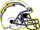Justin Herbert #10
 LAC Chargers, 3 years exp
GAM=17, PAYD=4739, PATD=25, RUCA=54, RUYD=147, RUTD=0, INT=10, FUM=3, FPPG=19.3, TFP=328
5TB Buccaneers, 23 years exp
GAM=17, PAYD=4694, PATD=25, RUCA=29, RUYD=-1, RUTD=1, INT=9, FUM=4, FPPG=18.7, TFP=318
6Lamar Jackson #13
 BAL Ravens, 5 years exp
GAM=12, PAYD=2242, PATD=17, RUCA=112, RUYD=764, RUTD=3, INT=7, FUM=2, FPPG=22.3, TFP=268
7Russell Wilson #3
 DEN Broncos, 10 years exp
GAM=15, PAYD=3524, PATD=16, RUCA=55, RUYD=277, RUTD=3, INT=11, FUM=2, FPPG=17.1, TFP=257
8Dak Prescott #12
 DAL Cowboys, 7 years exp
GAM=12, PAYD=2860, PATD=23, RUCA=45, RUYD=182, RUTD=1, INT=15, FUM=1, FPPG=20.4, TFP=245
9Trey Lance #5
 SF 49ers, 2 years exp
GAM=2, PAYD=194, PATD=0, RUCA=16, RUYD=67, RUTD=0, INT=1, FUM=0, FPPG=6.2, TFP=12
10Kyler Murray #1
 ARI Cardinals, 4 years exp
GAM=11, PAYD=2368, PATD=14, RUCA=67, RUYD=418, RUTD=3, INT=7, FUM=2, FPPG=20, TFP=221

## Top 10 Running Backs

(view all)
1Jonathan Taylor #34
 IND Colts, 3 years exp
GAM=11, RUCA=192, RUYD=861, RUTD=4, RETR=40, RECP=28, REYD=143, RETD=0, FUM=3, FPPG=10.8, TFP=118
2Austin Ekeler #30
 LAC Chargers, 6 years exp
GAM=17, RUCA=204, RUYD=915, RUTD=13, RETR=127, RECP=107, REYD=722, RETD=5, FUM=3, FPPG=15.6, TFP=266
3Derrick Henry #34
 TEN Titans, 7 years exp
GAM=16, RUCA=349, RUYD=1538, RUTD=13, RETR=41, RECP=33, REYD=398, RETD=0, FUM=3, FPPG=16.6, TFP=266
4Christian McCaffrey #34
 SF 49ers, 6 years exp
GAM=17, RUCA=244, RUYD=1139, RUTD=8, RETR=108, RECP=85, REYD=741, RETD=5, FUM=0, FPPG=15.6, TFP=266
5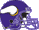Dalvin Cook #34
 MIN Vikings, 6 years exp
GAM=17, RUCA=264, RUYD=1173, RUTD=8, RETR=56, RECP=39, REYD=295, RETD=2, FUM=4, FPPG=11.7, TFP=199
6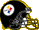Najee Harris #22
 PIT Steelers, 2 years exp
GAM=17, RUCA=272, RUYD=1034, RUTD=7, RETR=53, RECP=41, REYD=229, RETD=3, FUM=2, FPPG=10.7, TFP=182
7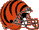Joe Mixon #34
 CIN Bengals, 6 years exp
GAM=14, RUCA=210, RUYD=814, RUTD=7, RETR=75, RECP=60, REYD=441, RETD=2, FUM=0, FPPG=12.8, TFP=180
8Leonard Fournette #23
 TB Buccaneers, 6 years exp
GAM=16, RUCA=189, RUYD=668, RUTD=3, RETR=83, RECP=73, REYD=523, RETD=3, FUM=0, FPPG=9.7, TFP=155
9Alvin Kamara #34
 NO Saints, 6 years exp
GAM=15, RUCA=223, RUYD=897, RUTD=2, RETR=77, RECP=57, REYD=490, RETD=2, FUM=4, FPPG=10.3, TFP=155
10Saquon Barkley #0
 NYG Giants, 5 years exp
GAM=16, RUCA=295, RUYD=1312, RUTD=10, RETR=76, RECP=57, REYD=338, RETD=0, FUM=0, FPPG=14.1, TFP=225

(view all)
1Cooper Kupp #80
 LAR Rams, 6 years exp
GAM=9, RETR=98, RECP=75, REYD=812, RETD=6, RUYD=52, RUTD=1, FUM=1, FPPG=14, TFP=126
2Justin Jefferson #80
 MIN Vikings, 3 years exp
GAM=17, RETR=184, RECP=128, REYD=1809, RETD=8, RUYD=24, RUTD=1, FUM=0, FPPG=14, TFP=237
3LV Raiders, 8 years exp
GAM=17, RETR=180, RECP=100, REYD=1516, RETD=14, RUYD=-1, RUTD=0, FUM=0, FPPG=13.9, TFP=236
4Tyreek Hill #80
 MIA Dolphins, 6 years exp
GAM=17, RETR=170, RECP=119, REYD=1710, RETD=7, RUYD=32, RUTD=1, FUM=0, FPPG=13.1, TFP=222
5Stefon Diggs #14
 BUF Bills, 8 years exp
GAM=16, RETR=154, RECP=108, REYD=1429, RETD=11, RUYD=-3, RUTD=0, FUM=0, FPPG=13, TFP=209
6CeeDee Lamb #85
 DAL Cowboys, 3 years exp
GAM=17, RETR=156, RECP=107, REYD=1359, RETD=9, RUYD=47, RUTD=0, FUM=0, FPPG=11.4, TFP=195
7Ja'Marr Chase #1
 CIN Bengals, 2 years exp
GAM=12, RETR=134, RECP=87, REYD=1046, RETD=9, RUYD=8, RUTD=0, FUM=2, FPPG=12.9, TFP=155
8A.J. Brown #80
 PHI Eagles, 4 years exp
GAM=17, RETR=145, RECP=88, REYD=1496, RETD=11, RUYD=0, RUTD=0, FUM=2, FPPG=12.4, TFP=212
9Michael Pittman #85
 IND Colts, 2 years exp
GAM=16, RETR=141, RECP=99, REYD=925, RETD=4, RUYD=30, RUTD=0, FUM=1, FPPG=7.3, TFP=118
10Tee Higgins #80
 CIN Bengals, 3 years exp
GAM=14, RETR=109, RECP=74, REYD=1029, RETD=7, RUYD=0, RUTD=0, FUM=0, FPPG=10.4, TFP=145

## Top 10 Tight Ends

(view all)
1Travis Kelce #87
 KC Chiefs, 10 years exp
GAM=17, RETR=152, RECP=110, REYD=1338, RETD=12, RUYD=5, RUTD=0, FUM=1, FPPG=12, TFP=204
2Darren Waller #85
 LV Raiders, 4 years exp
3Kyle Pitts #84
 ATL Falcons, 2 years exp
GAM=10, RETR=59, RECP=28, REYD=356, RETD=2, RUYD=0, RUTD=0, FUM=0, FPPG=4.8, TFP=48
4Mark Andrews #89
 BAL Ravens, 5 years exp
GAM=15, RETR=113, RECP=73, REYD=847, RETD=5, RUYD=8, RUTD=0, FUM=0, FPPG=7.7, TFP=116
5Dallas Goedert #85
 PHI Eagles, 5 years exp
GAM=12, RETR=69, RECP=55, REYD=702, RETD=3, RUYD=0, RUTD=0, FUM=1, FPPG=7.2, TFP=86
6Dalton Schultz #85
 DAL Cowboys, 5 years exp
GAM=15, RETR=89, RECP=57, REYD=577, RETD=5, RUYD=0, RUTD=0, FUM=1, FPPG=5.7, TFP=86
7George Kittle #85
 SF 49ers, 6 years exp
GAM=15, RETR=86, RECP=60, REYD=765, RETD=11, RUYD=0, RUTD=0, FUM=1, FPPG=9.4, TFP=140
8T.J. Hockenson #88
 MIN Vikings, 4 years exp
GAM=17, RETR=129, RECP=86, REYD=914, RETD=6, RUYD=0, RUTD=0, FUM=1, FPPG=7.4, TFP=125
9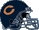Cole Kmet #80
 CHI Bears, 3 years exp
GAM=17, RETR=69, RECP=50, REYD=544, RETD=7, RUYD=9, RUTD=0, FUM=0, FPPG=5.7, TFP=97
10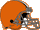David Njoku #85
 CLE Browns, 6 years exp
GAM=14, RETR=80, RECP=58, REYD=628, RETD=4, RUYD=-8, RUTD=0, FUM=1, FPPG=6, TFP=84

## Top 10 Kickers

(view all)
1Justin Tucker #9
 BAL Ravens, 11 years exp
GAM=17, MAFG=37, MIFG=6, MAXP=31, MIXP=1, FPPG=7.2, TFP=123
2Evan McPherson #19
 CIN Bengals, 2 years exp
GAM=16, MAFG=24, MIFG=5, MAXP=40, MIXP=5, FPPG=5.8, TFP=92
3Matt Gay #3
 LAR Rams, 4 years exp
GAM=17, MAFG=28, MIFG=2, MAXP=31, MIXP=1, FPPG=6.4, TFP=108
4Tyler Bass #2
 BUF Bills, 3 years exp
GAM=16, MAFG=27, MIFG=4, MAXP=48, MIXP=2, FPPG=7.2, TFP=115
5Harrison Butker #8
 KC Chiefs, 6 years exp
GAM=13, MAFG=18, MIFG=6, MAXP=38, MIXP=3, FPPG=5.5, TFP=71
6Greg Joseph #0
 MIN Vikings, 5 years exp
GAM=17, MAFG=26, MIFG=7, MAXP=40, MIXP=6, FPPG=5.4, TFP=91
7Jake Elliott #8
 PHI Eagles, 6 years exp
GAM=16, MAFG=20, MIFG=3, MAXP=51, MIXP=2, FPPG=6.2, TFP=100
8Daniel Carlson #7
 LV Raiders, 5 years exp
GAM=16, MAFG=34, MIFG=3, MAXP=35, MIXP=1, FPPG=7.9, TFP=127
9Robbie Gould #9
 SF 49ers, 18 years exp
GAM=17, MAFG=27, MIFG=5, MAXP=50, MIXP=1, FPPG=6.8, TFP=115
10Jason Sanders #3
 MIA Dolphins, 5 years exp
GAM=17, MAFG=26, MIFG=6, MAXP=41, MIXP=3, FPPG=5.8, TFP=98

## Top 10 Defenses/Special Teams

(view all)
1Los Angeles Rams
GAM=17, FREC=6, INT=16, SACK=38, DTD=1, PA=384, FPPG=5.2, TFP=88
2Denver Broncos
GAM=17, FREC=8, INT=15, SACK=36, DTD=0, PA=359, FPPG=5.1, TFP=86
3Pittsburgh Steelers
GAM=17, FREC=3, INT=20, SACK=40, DTD=1, PA=346, FPPG=5.4, TFP=92
4San Francisco 49ers
GAM=17, FREC=10, INT=20, SACK=44, DTD=3, PA=277, FPPG=7.2, TFP=122
5New Orleans Saints
GAM=17, FREC=7, INT=7, SACK=48, DTD=1, PA=345, FPPG=4.8, TFP=82
6Buffalo Bills
GAM=17, FREC=10, INT=17, SACK=40, DTD=1, PA=286, FPPG=6.4, TFP=108
7Cleveland Browns
GAM=17, FREC=9, INT=11, SACK=34, DTD=3, PA=381, FPPG=5.4, TFP=92
8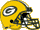Green Bay Packers
GAM=17, FREC=7, INT=17, SACK=34, DTD=2, PA=371, FPPG=5.8, TFP=98
9Tampa Bay Buccaneers
GAM=17, FREC=10, INT=10, SACK=45, DTD=1, PA=358, FPPG=5.4, TFP=91
10Indianapolis Colts
GAM=17, FREC=11, INT=10, SACK=44, DTD=2, PA=427, FPPG=5.8, TFP=98